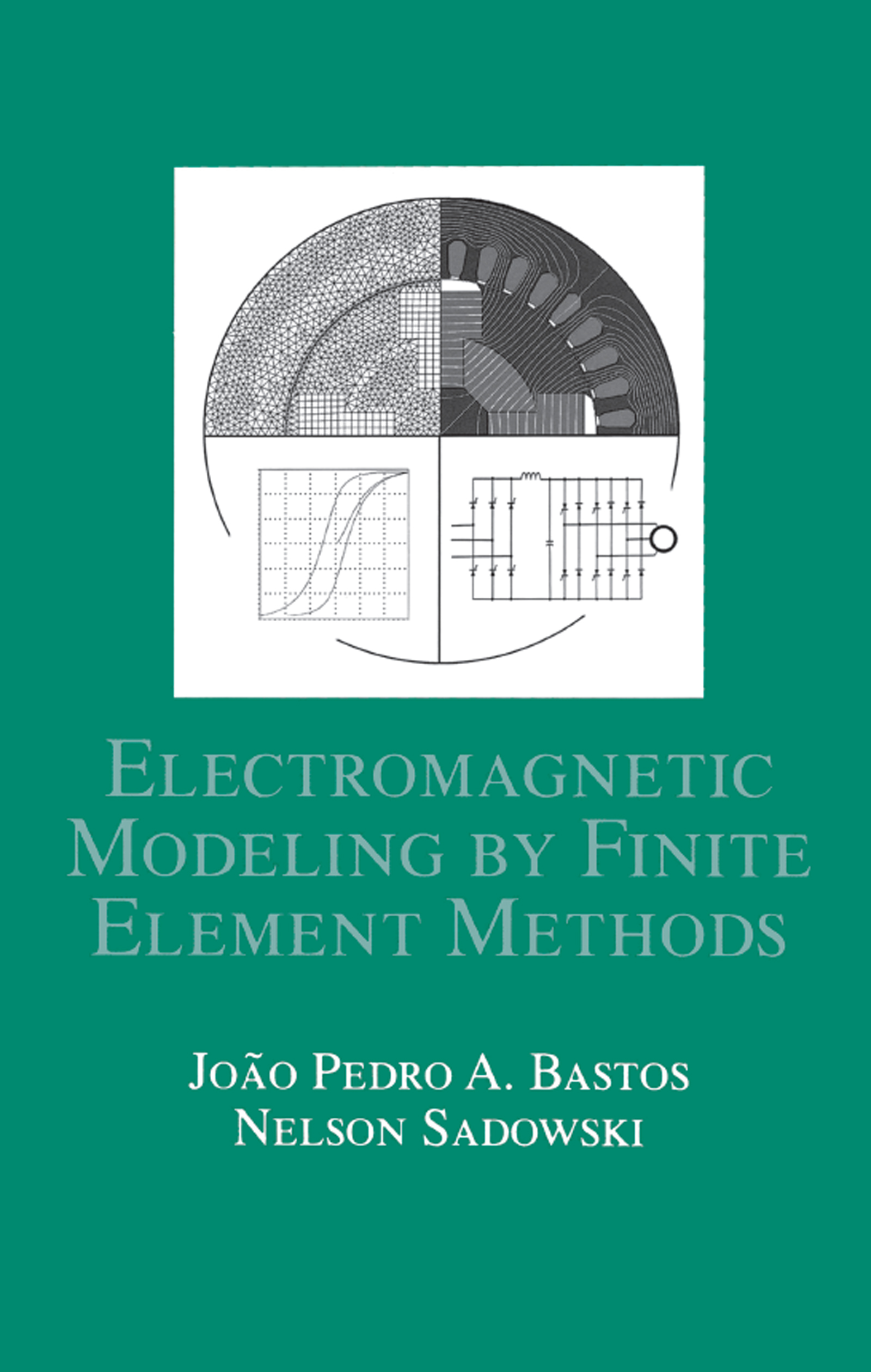Electromagnetic Modeling by Finite Element Methods

1st Edition

CRC Press

510 pages

Purchasing Options:\$ = USD
Hardback: 9780824742690
pub: 2003-04-01
SAVE ~\$29.00
\$145.00
\$116.00
x

FREE Standard Shipping!

Description

Unlike any other source in the field, this valuable reference clearly examines key aspects of the finite element method (FEM) for electromagnetic analysis of low-frequency electrical devices. The authors examine phenomena such as nonlinearity, mechanical force, electrical circuit coupling, vibration, heat, and movement for applications in the electrical, mechanical, nuclear, aeronautics, and transportation industries.

Electromagnetic Modeling by Finite Element Methods offers a wide range of examples, including torque, vibration, and iron loss calculation; coupling of the FEM with mechanical equations, circuits, converters, and thermal effects; material modeling; and proven methods for hysteresis implementation into FEM codes.

Providing experimental results and comparisons from the authors' personal research, Electromagnetic Modeling by Finite Element Methods supplies techniques to implement FEM for solving Maxwell's equations, analyze electrical and magnetic losses, determine the behavior of electrical machines, evaluate force distribution on a magnetic medium, simulate movement in electrical machines and electromagnetic devices fed by external circuits or static converters, and analyze the vibrational behavior of electrical machines.

PREFACE

MATHEMATICAL PRELIMINARIES

Introduction

The Vector Notation

Vector Derivation

The Divergence

The Rotational

Second-Order Operators

Application of Operators to More than One Function

Expressions in Cylindrical and Spherical Coordinates

MAXWELL EQUATIONS, ELECTROSTATICS, MAGNETOSTATICS, AND MAGNETODYNAMIC FIELDS

Introduction

The EM Quantities

Local Form of the Equations

The Anisotropy

The Approximation of Maxwell's Equations

The Integral Form of Maxwell's Equations

Electrostatic Fields

Magnetostatic Fields

Magnetodynamic Fields

BRIEF PRESENTATION OF THE FINITE ELEMENT METHOD

Introduction

The Galerkin Method - Basic Concepts

A First-Order Finite Element Program

Generalization of the Finite Element Method

Numerical Integration

Some 2D Finite Elements

Coupling Different Finite Elements

Calculation of Some Terms in the Field Equation

A Simplified 2D Second-Order Finite Element Program

THE FINITE ELEMENT METHOD APPLIED TO 2D ELECTROMAGNETIC CASES

Introduction

Some Static Cases

Application to 2D Eddy Current Problems

Axi-Symmetric Application

Advantages and Limitation of 2D Formulations

Non-Linear Applications

Geometric Repetition of Domains

Thermal Problems

Voltage-Fed Electromagnetic Devices

Static Examples

Dynamic Examples

COUPLING OF FIELD AND ELECTRICAL CIRCUIT EQUATIONS

Introduction

Electromagnetic Equations

Equations for Different Conductor Configurations

Connections Between Electromagnetic Devices and External Feeding Circuits

Examples

MOVEMENT MODELING FOR ELECTRICAL MACHINES

Introduction

The Macro-Element

The Moving Band

The Skew Effect in Electrical Machines Using 2D Simulation

Examples

INTERACTION BETWEEN ELECTROMAGNETIC AND MECHANICAL FORCES

Introduction

Methods Based on Direct Formulations

Methods Based on the Force Density

Electrical Machine Vibrations Originated by Magnetic Forces

Example of Coupling Between the Field and Circuit Equations, Including Mechanical Transients

IRON LOSSES

Introduction

Eddy Current Losses

Hysteresis

Anomalous or Excess Losses

Total Iron Losses

The Jiles-Atherton Model

The Inverse Jiles-Atherton Model

Including Iron Losses in Finite Element Calculations

BIBLIOGRAPHY

INDEX